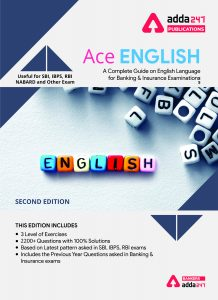Latest Banking jobs   »   English Quizzes, for SBI PO, Clerk...

# English Quizzes, for SBI PO, Clerk Prelims 2021 – 27th June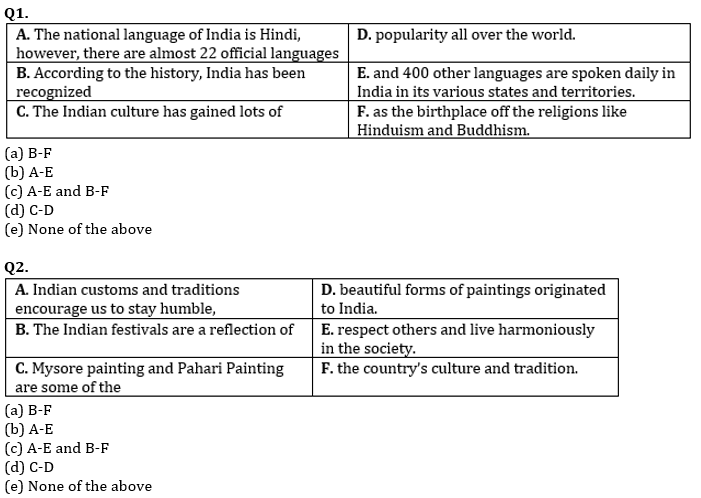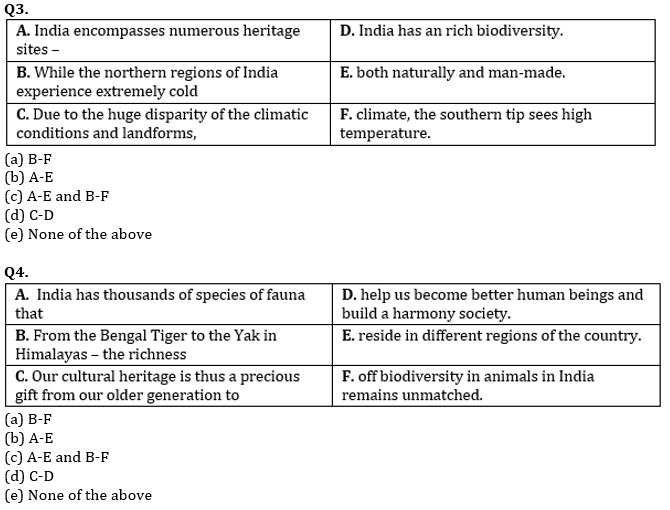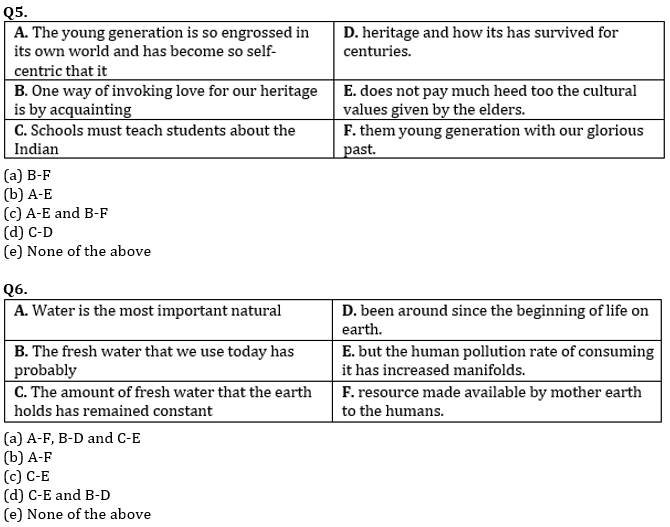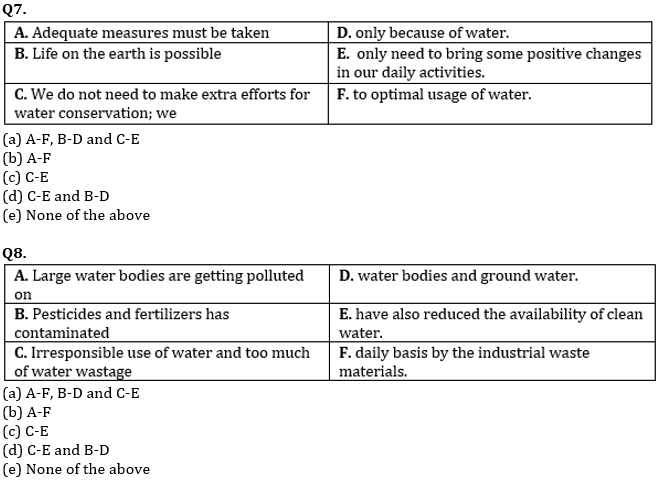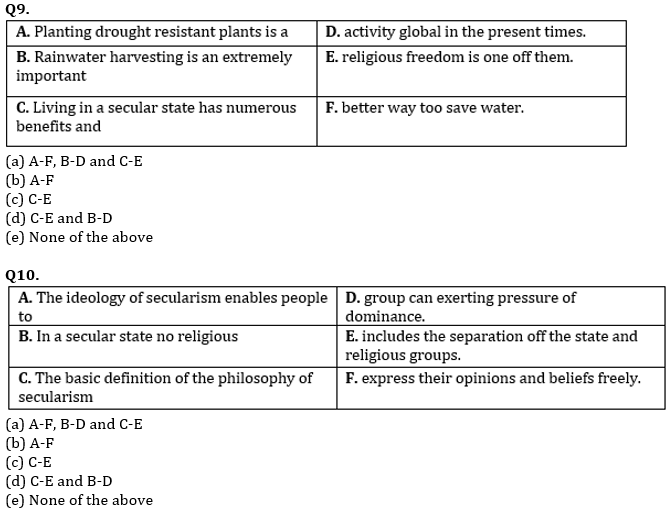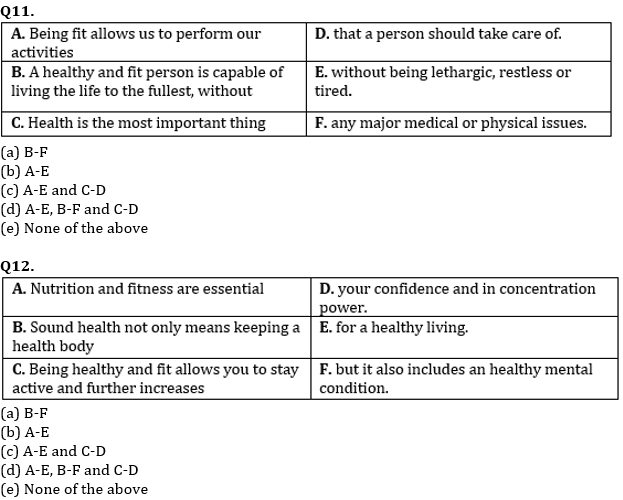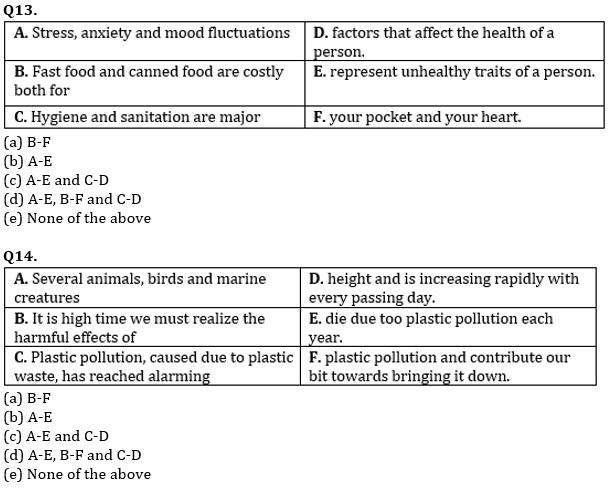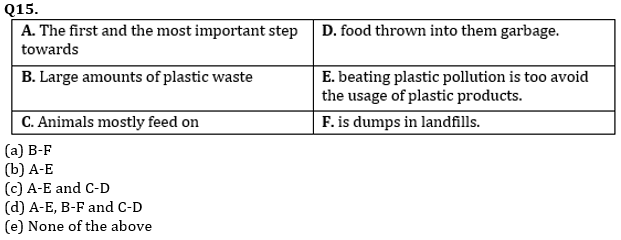Solutions

S1. Ans.(d)
Sol. ‘C-D’ is the correct combination here and makes a meaningful sentence.

S2. Ans.(c)
Sol. ‘A-E’ and ‘B-F’ are the correct combinations here and make meaningful sentences.

S3. Ans.(a)
Sol. ‘B-F’ is the correct combination here and makes a meaningful sentence.

S4. Ans.(b)
Sol. ‘A-E’ is the correct combination here and makes a meaningful sentence.

S5. Ans.(e)
Sol. None of the above

S6. Ans.(a)
Sol. ‘A-F’, ‘B-D’ and ‘C-E’ are the correct combinations here and make meaningful sentences.

S7. Ans.(d)
Sol. ‘B-D’ and ‘C-E’ are the correct combinations here and make meaningful sentences.

S8. Ans.(c)
Sol. ‘C-E’ is the correct combination here and makes a meaningful sentence.

S9. Ans.(e)
Sol. None of the above

S10. Ans.(b)
Sol. ‘A-F’ is the correct combination here and makes a meaningful sentence.

S11. Ans.(c)
Sol. ‘A-E’ and ‘C-D’ are the correct combinations here and make meaningful sentences.

S12. Ans.(b)
Sol. ‘A-E’ is the correct combination here and makes a meaningful sentence.

S13. Ans.(d)
Sol. ‘A-E’, ‘B-F’ and ‘C-D’ are the correct combinations here and make meaningful sentences.

S14. Ans.(a)
Sol. ” ‘B-F’ is the correct combination here and makes a meaningful sentence. In the case of ‘A-E’ it should be ‘to’ instead of ‘too’ and in the case of ‘C-D’ it should be ‘alarming heights’.”

S15. Ans.(e)
Sol. None of the above